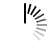# Sort strings in Alphanumeric sequence

A list of given strings is sorted in alphanumeric order or Dictionary Order. Like for these words: Apple, Book, Aim, they will be sorted as Aim, Apple, Book.If there are some numbers, they can be placed before the alphabetic strings.

#### Input and Output

```Input:
A list of strings: Ball Apple Data Area 517 April Man 506
Output:
Strings after sort: 506 517 Apple April Area Ball Data Man```

#### Algorithm

`sortStr(strArr, n)`

Input: The list of all strings, number of elements.

Output: Strings in alphanumeric sorted order.

```Begin
for round := 1 to n-1, do
for i := 0 to n-round, do
res := compare str[i] and str[i+1]       //either +ve, or –ve or 0
if res > 0, then
swap str[i] and str[i+1]
done
done
End```

#### Source Code (C++)

```#include<iostream>
#define N 8
using namespace std;

void display(int n, string str[]) {
for(int i = 0; i<n; i++)
cout << str[i] << " ";         //print the string from array
cout << endl;
}

void sortStr(int n, string str[]) {
int i, round, res;
for(round = 1; round<n; round++)
for(i = 0; i<n-round; i++) {
res = str[i].compare(str[i+1]);
if(res > 0)
swap(str[i], str[i+1]);//swap strings
}
}

main() {
string str[N] = {"Ball", "Apple", "Data", "Area", "517", "April", "Man", "506"};
cout << "Strings before sort:"<< endl;
display(N, str);
sortStr(N, str);
cout << "Strings after sort:"<<endl;
display(N, str);
}```

#### Output

```Strings before sort:
Ball Apple Data Area 517 April Man 506
Strings after sort:
506 517 Apple April Area Ball Data Man```